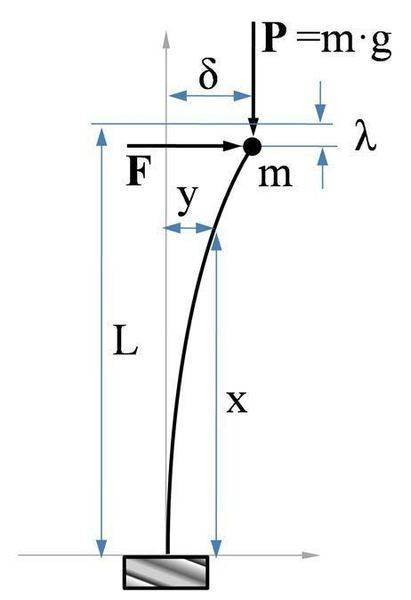# Column buckling under axial+transverse combined load

Dear experts,

Do you know the buckling load of a column (one end clamped one end free) under axial compressive and transverse lateral forces (applied combined at top free end)?

Does the lateral load reduce the critical load or just reduces the stiffness of the column?

Would you help me?
-Thanks!

Nidum
Gold Member
What do you think ?

Try drawing some diagrams and see if you can see intuitively what is likely to happen .

${U_{(\delta )}} = {V_{(\delta )}} \\ \frac{{4{P^2}{\delta ^2}L}}{{15EI}} = F\delta + \frac{{2{\delta ^2}P}}{{3L}}$